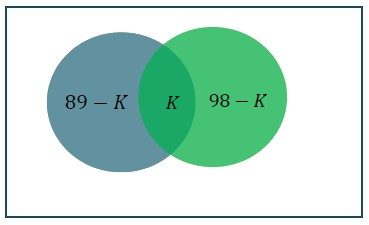# In a hospital, 89% has disease A and 98% has disease B, K% is the number of patients who has both, then K can’t be the subset of ____

Solution:

Answer: Least k =87, Max k = 89Let total =100

n(A)=89, n(B)=98

max (n(A),n(B)) ≤ n(AUB) ≤ 100

98 ≤ n(A)+n(B)-n(A n B) ≤ 100

=> 98 ≤ 98 + 89- k ≤ 100

Least k =87

Max k = 89(0)(0)